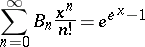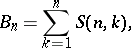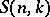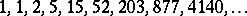# Bell numbers

(diff) ← Older revision | Latest revision (diff) | Newer revision → (diff)

The Bell numbersare given byor byAlso,whereare Stirling numbers (cf. Combinatorial analysis) of the second kind, so thatis the total number of partitions of an-set.

They are equal to.

The name honours E.T. Bell.

How to Cite This Entry:
Bell numbers. Encyclopedia of Mathematics. URL: http://encyclopediaofmath.org/index.php?title=Bell_numbers&oldid=14335
This article was adapted from an original article by N.J.A. Sloane (originator), which appeared in Encyclopedia of Mathematics - ISBN 1402006098. See original article